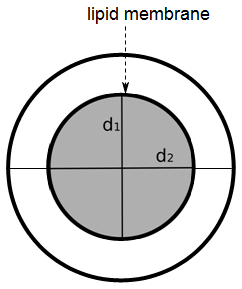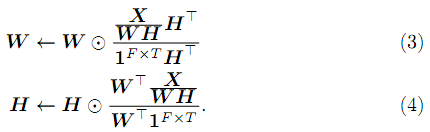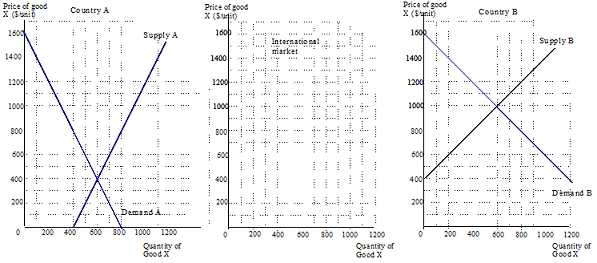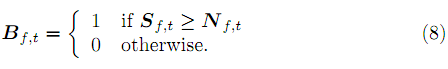+61-413 786 465

info@mywordsolution.com

## Engineering

 Civil Engineering Chemical Engineering Electrical & Electronics Mechanical Engineering Computer Engineering Engineering Mathematics MATLAB Other Engineering Digital Electronics Biochemical & Biotechnology

Assignment - Machine Learning for Signal Processing

Problem 1: Instantaneous Source Separation

1. As you might have noticed from my long hair, I've got a rock spirit. However, for this homework I dabbled to compose a piece of music that is jazzy. The title of the song is boring: Homework 3.

2. From x_ica_1.wav to x_ica 20.wav are 20 recordings of my song, Homework 3. Each recording has N time domain samples. In this music there are K unknown number of musical sources played at the same time. In other words, as I wanted to disguise the number of sources, I created unnecessarily many recordings of this simple music. This can be seen as a situation where the source was mixed up with a 20 × K mixing matrix A to the K sources to create the 20 channel mixture:3. But, as you've learned how to do source separation using ICA, you should be able to separate them out into K clean speech sources.

4. First, you don't like the fact that there are too many recordings for this separation problem, because you have a feeling that the number of sources is a lot smaller than 20. So, you decided to do a dimension redcution first, before you actually go ahead and do ICA. For this, you choose to perform PCA with whitening option. Apply your PCA algorithm on your data matrix X, a 20 × N matrix. Don't forget to whiten the data. Make a decision as to how many dimensions to keep, which will correspond to your K. Hint: take a very close look at your eigenvalues.

5. On your whitened/dimension reduced data matrix Z (K ×N), apply ICA. At every iteration of the ICA algorithm, use these as your update rules:

ΔW ← (NI - g(Y)f(Y)')W

W ← W + ρΔW

Y ← WZ

where

W: The ICA unmixing matrix you're estimating

Y: The K × N source matrix you're estimating

Z: Whitened/dim reduced version of your input (using PCA)

g(x): tanh(x)

f(x): x3

ρ: learning rate

N: number of samples

6. Enjoy your separated music. Submit your separated .wav files, source code, and the convergence graph.

7. Implementation notes: Depending on the choice of the learning rate the convergence of the ICA algorithm varies. But I always see the convergence in from 5 sec to 90 sec in my iMac.

Problem 2: Single-channel Source Separation

1. You can use a variety of error functions for the NMF algorithm. An interesting one is to see the matrices as a probabilistic distribution though not normalized. I actually prefer this one than the one I introduced in L6 S35. From this, you can come up with this error function:

ε(X||WH) = ∑i,j Xi,j log(Xi,j/X^i,j) - Xi,j + X^i,j,  (2)

where Xˆ = WH. I wouldn't bother you with a potential question that might ask you to derive the update rules. Instead, I'll just give them away here:Note that 1FxT is a matrix full of ones, whose size is F x T, where F and T are the number of rows and columns of your input matrix X, respectively. Please note that, once again, we have multiplicative update rules. Therefore, once you initialized your parameter matrices W and H with nonnegative random values, you can keep their non-negativity as long as your input matrix X is nonnegative as well.

2. trs.wav is a speech signal of a speaker. Load it and convert it into the time-frequency domain by using STFT. For STFT, take a frame of 1024 samples with 50% overlap. Use Hann windows. This will give 1024 × 990 complex-valued matrix (You could get slightly more or less columns than mine depending on your STFT setup). From homework 1 problem 1, you should have noticed that this kind of matrix has complex conjugacy: bottom half of the spectrogram is a mirrored version of the upper half. This is because of the fact that cos(θ) = cos(2π - θ) (the real part) and sin(θ) = - sin(2π - θ) (the imaginary part). Discard the complex conjugate part (the bottom half). So eventually it will be an 513 × 990 matrix. Later on in this problem when you recover the time domain signal out of this, you can easily recover the discarded half from the existing half so that you can do inverse-DFT on the column vector of full 1024 points. Hint: Why 513, not 512? Create a very short random signal with 16 samples, and do a DFT transform to convert it into a spectrum of 16 complex values. Check out their complex coefficients to see why you need 1024/2 + 1, not 1024/2.

3. Take the magnitudes of this matrix. Let's call these magnitudes S, which is nothing but a matrix of nonnegative elements. Learn an NMF model out of this such as S ≈ WSHS using the update rules (3) and (4). You know, WS is a set of basis vectors. If you choose to learn this NMF model with 30 basis vectors, then WS ∈ R513×30+, where R+ is a set of nonnegative real numbers. You're going to use WS for your separation.

4. Repeat this process for your another training signal trn.wav. Learn another NMF model from trn.wav, which is another training signal for your noise. From this get WN.

5. x_nmf.wav is a noisy speech signal made of the same speaker's different speech and the same type of noise you saw. By using our third NMF model, we're going to denoise this one. Load this signal and convert it into a spectrogram X ∈ C513×131. Let's call its magnitude spectrogram Y = |X| ∈ R513×131+. Your third NMF will learn this approximation:

Y ≈ [WSWN]H. (5)

What this means is that for this third NMF model, instead of learning new basis vectors, you reuse the ones you trained from the previous two models as your basis vectors for testing: W = [WSWN]. As you are very sure that the basis vectors for your test signal should be the same with the ones you trained from each of the sources, you initialize your W matrix with the trained ones and don't even update it during this third NMF. Instead, you learn a whole new H ∈ R60×131+ that tells you the activation of the basis vectors for every given time frame. Implementation is simple. Skip the update for W. Update H by using W = [WSWN] and Y. Repeat. Note: You're doing a lot of element-wise division, so be careful not to divide by zero. To prevent this, I tend to add a very small value (e.g. eps in MATLAB) to every denominator element in the update rule.

6. Because WSH(1:30,:) can be seen as the speech source estimate, you can also create a magnitude masking matrix out of it:

M¯ = WSH(1:30,:)/(WSH(1:30,:) + WNH(31:60,:)) = WSH(1:30,:)/[WSWN]H. (6)

Use this masking matrix to recover your speech source, i.e. Sˆ = M¯X. Recover the bottom 511 rows by using the conjugacy discussed in 2. Invert back to the time domain. Listen to it to see if the noise is suppressed.

Submit the wav file and source code.

Problem 3: Motor Imagery

1. eeg.mat has the training and testing samples and their labels. Use them to replicate my BCI classification experiments in Module 5 (not the entire lecture, but from S3 to S8 and S37). But, instead of PCA dimension reduction, we're going to use locality sensitive hashing to extract binary features. Also, instead of naive Bayes, we'll do kNN classification.

2. For the kNN classification, for every test example you have to find the K nearest neighbors from 112 training samples. You're lucky. This kNN search is not a big deal, because your training set is tiny. However, as your professor I have to help you guys be prepared for your future big data projects at your fancy new job after your graduation (don't forget me). So, I'm giving you this homework assignment that will help you come up with a speed-up technique for the kNN search. It's not that complicated.

3. Come up with a random projection matrix A ∈ RL×M, where M denotes the number of dimensions of your data samples (5 rows of your magnitude STFT, vectorized), 255, and L is the new dimension after this random projection. So, you're replacing PCA with this random projection. Note that L doesn't always have to be smaller than M. Although A is a matrix with random values, you should make sure that the row vectors (the projection vectors) should be unit vectors, i.e. ||Ai,:||2 = 1. Make sure of it.

4. Do the random projection and take the sign of the result (element-wise), so that your random projection produces a bunch of +1's and -1's:

Y = sign(AZ1:M,:) (7)

5. Use this A matrix and the sign function wrapper to convert your 255 dimensional TRAINING samples into L-dimensional bipolar binary hash codes. Ditto for the TEST samples (use the same A matrix). If you're not comfortable with bipolar binaries, you can turn them into 0-1 binaries by replacing -1's with 0's. It's up to you.

6. Do your kNN classification using these binary version of your data samples. Note that you can compare the test bit string with the bit strings of the training data by using the Hamming distance, instead of the Eucleadian distance between the original real-valued vectors. You know your computer prefers binary values, right? This way you can speed up the comparison, and eventually the kNN search (although you might not be able to see the actual speed-up due to the too small dataset size and your script language that doesn't exploit binary variables). Report your classification results with different choice of K, L and M. I think a table of accuracies will be great. You don't have to submit all the intermediate plots. What I need to see is the accuracies and your source code.

7. Compare your results with mine in M5 S37. You're classifier is based on binary variables and bitwise operations, so you must have expected a big performance drop. How do you like it eventually? Report your classification accuracy. Submit your code and your discussion.

Problem 4: kNN Source Separation

1. Prepare your S and N matrices by using the procedure in 2.2-2.4, but without NMF, i.e. they are the input magnitude spectrogram to your NMF. This time, let's create another matrix G, the magnitude spectrogram of trs.wav+trn.wav. Also, let's create another matrix called Ideal Binary Masks (IBM), B defined as follows:My hope is that S ≈ BG. This sounds a bit too rough, but it actually works well, because if you take a look at the local bins, they are dominated by one of the sources, not really all of them due to the sparsity of the sources in the TF domain. If you can't believe in me, go ahead and convert S back to the time domain (with proper phase information) to check out the sound, although I don't require it.

2. Load x_nmf.wav and convert it into X and Y like in 2.5. We're not doing NMF on this though. This time, we compare each column of Y with all columns in G to find out k nearest neighbors. Then, for t-th given test frame, we have a list of indices to the k nearest frames in G, e.g. {i1, i2, · · · , ik}, where i1, i2, · · · , ik ∈ {1, · · · , 990}. For the comparison, I used Euclidean distance for no good reason, but you can choose a different (more proper) one if you want.

3. What we want to do with this is to predict the IBM of the test signal, D ∈ B513×131. By using the nearest neighbor indices, I can collect the IBM vectors from B, i.e. {B:,i1, B:,i2, · · · , B:,ik}. Since Y:,t is very similar to {G:,i1, G:,i2, · · · , G:,ik}, I hope that my D:,t should be similar to {B:,i1, B:,i2, · · · , B:,ik}. How do we consolidate them though? Let's try median of them, because that'll give you one if one is the majority, and vice versa:

D:,t = median({B:,i1, B:,i2, · · · , B:,ik}). (9)

4. Repeat this for all your test frames (131 frames in my STFT) and predict the full D matrix. Recover the complex-valued spectrogram of your speech by masking the input: Sˆtest = DX. Convert it back to the time domain. Listen to it. Is the noise suppressed? Submit this wavefile as well as your code.

Attachment:- Assignment Files - Advanced Machine Learning.rar

Mechanical Engineering, Engineering

• Category:- Mechanical Engineering
• Reference No.:- M93125120
• Price:- \$160

Guranteed 48 Hours Delivery, In Price:- \$160

Have any Question?

## Related Questions in Mechanical Engineering

### Problem on shaft -assuming no frictional losses a torque of

Problem on Shaft - Assuming no frictional losses, a torque of T = 750lb·in is applied through the shaft of gear F in order to drive the roller chain at point B at a constant speed. The chain sprocket has a pitch diameter ...

### 1 explain the term critical equipment and provide two clear

1. Explain the term "critical equipment" and provide two clear examples of machinery which falls into this category on board a twin screw motor vessel. 2. Explain the process of Charpy lmpact Testing including sample mar ...

### Problem -a long pipe od 1413 mm id 1318 mm kp 20 wmk

Problem - A long pipe (OD = 141.3 mm, ID =131.8 mm, k p = 20 W/m.K) supplies hot pressurized liquid water at 400 K to a heater in a factory. The pipe has an insulation thickness of 100 mm. A new efficient heater replaces ...

### Question - truss structureconsider the three membered

Question - Truss Structure Consider the three membered planar truss structure shown in the figure below. All members of the truss have identical square cross-sectional area (A) of 25 mm x 25 mm, and Youngs Modulus (E) = ...

### Materials behaviour from atoms to bridges assignment -

Materials Behaviour from Atoms to Bridges Assignment - Distributed loads and static equilibrium (Please note: you should show your steps with necessary figures) Q1. Two beam sections are jointed at C and supported at A b ...

### Fluid mechanics assignment - finite control volume analysis

Fluid Mechanics Assignment - Finite Control Volume Analysis and Dimensional Analysis Q1. The wind blows through a 7ft × 10ft garage door opening with a speed of 5 ft/s as shown in the figure. Determine the average speed, ...

### Assignment -q1 explain the difference between the

Assignment - Q1. Explain the difference between the metacentric height of a ship during 'Partially Afloat condition and 'Free Floating' condition; aid a sketch to support your answer. Q2. With the aid of sketches, explai ...

### Structural mechanics questions -q1 a 150-lb bucket is

Structural Mechanics Questions - Q1. A 150-lb bucket is suspended from a cable on the wooden frame. Determine the resultant internal loadings on the cross section at D, and at E. Q2. The shaft is supported at its ends by ...

### Questions -q1 a qualitative estimate of the effect of a

Questions - Q1. A qualitative estimate of the effect of a wind-tunnel contraction (Figure) on turbulent motion can be obtained by assuming that the angular momentum of eddies does not change through the contraction. Let ...

### Life cycle assessmentfor your chosen service eg white board

LIFE CYCLE ASSESSMENT For your chosen service (e.g. white board markers), identify two alternatives of getting the service (e.g. brand A, brand B). Choose the most environmentally friendly option by conducting life cycle ...

• 13,132 Experts

## Looking for Assignment Help?

Start excelling in your Courses, Get help with Assignment

Write us your full requirement for evaluation and you will receive response within 20 minutes turnaround time.

### Why might a bank avoid the use of interest rate swaps even

Why might a bank avoid the use of interest rate swaps, even when the institution is exposed to significant interest rate

### Describe the difference between zero coupon bonds and

Describe the difference between zero coupon bonds and coupon bonds. Under what conditions will a coupon bond sell at a p

### Compute the present value of an annuity of 880 per year

Compute the present value of an annuity of \$ 880 per year for 16 years, given a discount rate of 6 percent per annum. As

### Compute the present value of an 1150 payment made in ten

Compute the present value of an \$1,150 payment made in ten years when the discount rate is 12 percent. (Do not round int

### Compute the present value of an annuity of 699 per year

Compute the present value of an annuity of \$ 699 per year for 19 years, given a discount rate of 6 percent per annum. As